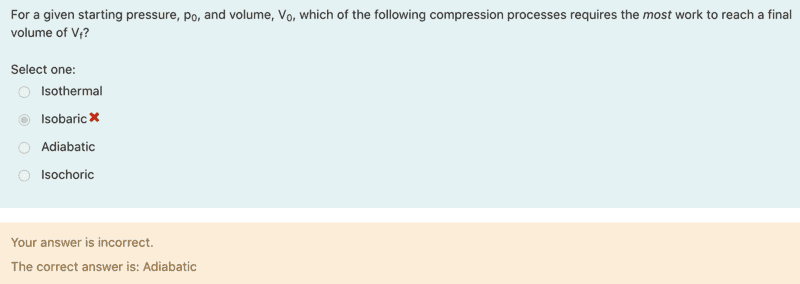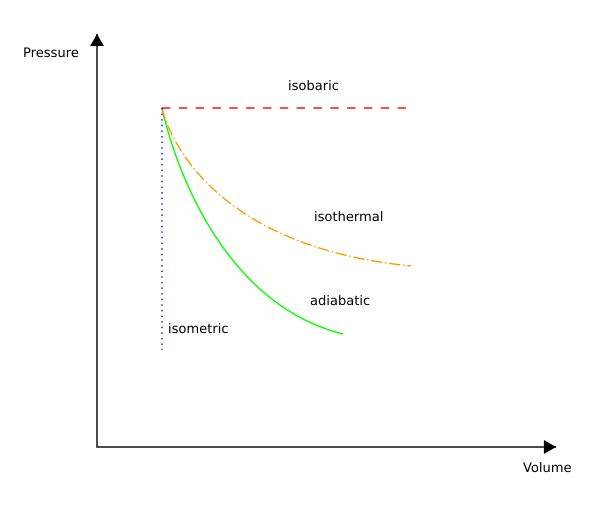# Thermodynamic Process that Produces the Most Work

sd17
Homework Statement:
Do you think my lecturer is correct in saying this is the correct answer? Question: For a given starting pressure and volume, which of the following compression processes requires the most work to reach a final volume: Isothermal, isobaric, adiabatic, isochoric?
Relevant Equations:
delta(U) = Q - W•Delta2

Staff Emeritus
Homework Helper
Gold Member
You have just posted the model answer. In the homework sections you are required to provide your own arguments and reasoning. So you seem to disagree with the model answer, why?

sd17
You have just posted the model answer. In the homework sections you are required to provide your own arguments and reasoning. So you seem to disagree with the model answer, why?
The reason I chose isobaric is because of the diagrams that I have seen in textbooks and other sources where it appears that the area under the curve is greater than other processes.•Delta2
willem2
The reason I chose isobaric is because of the diagrams that I have seen in textbooks and other sources where it appears that the area under the curve is greater than other processes.
The question is about compression, you might want to draw that diagram again, where all the processes start out at the same volume and pressure, and the volume decreases, while the pressure increases.

•Lnewqban, BvU, Delta2 and 1 other person
Staff Emeritus
Homework Helper
Gold Member
To build a bit upon that. In the diagram you have attached, if you consider the curves curves for compressions (i.e., going right to left along the curves), then they all end at the same volume and pressure. However, the problem statement tells you that the processes should all start at the same volume and pressure, which makes the analysis different.

It is however questionable that the problem statement calls an isochoric process a compression process since volume is constant in such a process by definition.

•Lnewqban
sd17
The question is about compression, you might want to draw that diagram again, where all the processes start out at the same volume and pressure, and the volume decreases, while the pressure increases.
Thank you for your input I am now able to see that adiabatic is the correct answer.

•vela, Lnewqban and Delta2
sd17
To build a bit upon that. In the diagram you have attached, if you consider the curves curves for compressions (i.e., going right to left along the curves), then they all end at the same volume and pressure. However, the problem statement tells you that the processes should all start at the same volume and pressure, which makes the analysis different.

It is however questionable that the problem statement calls an isochoric process a compression process since volume is constant in such a process by definition.
Thank you for your reply I am now able to understand the reasoning behind the correct answer.

•Lnewqban
Homework Helper
Gold Member
Homework Statement:: Do you think my lecturer is correct in saying this is the correct answer? Question: For a given starting pressure and volume, which of the following compression processes requires the most work to reach a final volume: Isothermal, isobaric, adiabatic, isochoric?
Relevant Equations:: delta(U) = Q - W
Am I the only one to think that the statement of the problem is ambiguous? I am always wary when I see "work" mentioned without reference as to who is doing the work on whom. Prepositions are particularly important in this case. If we compare the isobaric and adiabatic processes, the magnitude of the work done is positive regardless of whether the work is done by the gas or on the gas by the environment. The isobaric process has more area under the curve which makes the magnitude of the work (amount of Joules) exchanged with the environment greater than the adiabatic.

For a compression, the work done by the gas on the environment is always negative. A negative number with a larger magnitude is smaller, so the answer would be "adiabatic" because it is closer to zero than "isobaric". However, the reverse would be true for the work done on the gas by the environment and the correct answer would be "isobaric". Does "most" work in the statement of the problem mean "more Joules exchanged" or does it mean "the larger of two negative numbers"?

In my mind, the question is ambiguous because of the lack of prepositions. The only clue that we have is OP's relevant equation of the first law. One can tell by the negative sign that ##W## is the work done by the gas on the environment. However, many textbooks write the first law with a plus sign in front of ##W## which implicitly defines it as the work done on the gas by the environment. I think that in this case, the proper use of prepositions would have made the correct answer independent of which convention one uses for the first law.

Staff Emeritus
Homework Helper
Gold Member
The isobaric process has more area under the curve
No it does not. Not if drawn in accordance with the problem statement that specifies equal pressure and volume before compression, ie, the curves should coincide on the right side. The isobaric curve will lie below all the others.

•Delta2
•